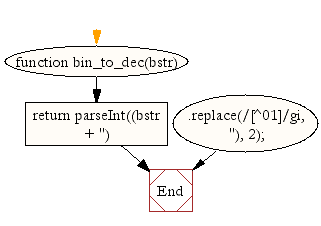# JavaScript: Convert a binary number to a decimal number

## JavaScript Math: Exercise-2 with Solution

Write a JavaScript function to convert a binary number to a decimal number.

Test Data: console.log(bin_to_dec('110011')); console.log(bin_to_dec('100')); 51 4

Pictorial Presentation:Sample Solution:-

HTML Code:

``````<!DOCTYPE html>
<html>
<head>
<meta charset="utf-8">
<title>Binary number to a decimal number</title>
</head>
<body>

</body>
</html>
```
```

JavaScript Code:

``````function bin_to_dec(bstr) {
return parseInt((bstr + '')
.replace(/[^01]/gi, ''), 2);
}
console.log(bin_to_dec('110011'));
console.log(bin_to_dec('100'));
```
```

Sample Output:

```51
4
```

Flowchart:Live Demo:

See the Pen javascript-math-exercise-2 by w3resource (@w3resource) on CodePen.

Improve this sample solution and post your code through Disqus

What is the difficulty level of this exercise?

﻿

## JavaScript: Tips of the Day

Convert tabs to spaces, where each tab corresponds to count spaces

Example:

```const tips_expandTabs = (str, count) => str.replace(/\t/g, ' '.repeat(count));
console.log(tips_expandTabs('\t\tw3resource', 4));
```

Output:

```"        w3resource"
```